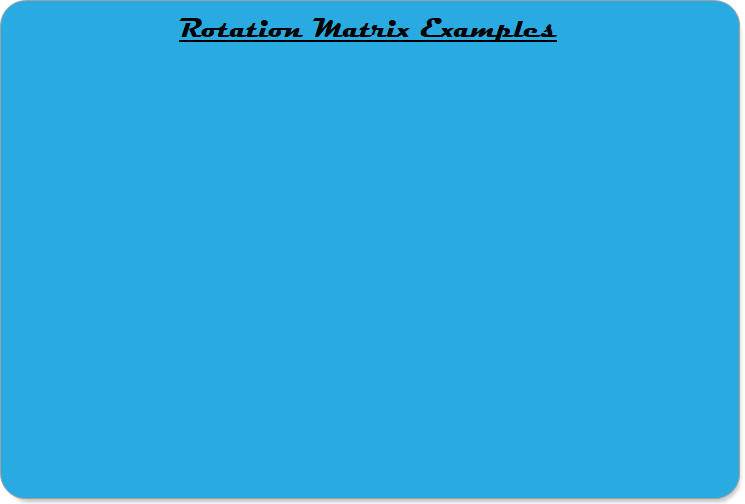Robotics 1
Rotation MatricesThis video introduces the concept of 'Rotation Matrices' as a way to represent the rotation, or orientation, of one coordinate frame relative to another.  The three rotation matrices (rotation around X, Y, and Z) are given, and the derivation of the rotation around the Z axis shown. This video also shows how any rotations can be accomplished by stringing together rotations around X, Y, and Z, and multiplying the corresponding matrices.This video teaches how to compute rotation matrices in Python, and discusses the meaning of the numbers calculated relative to the manipulator.This video shows five examples of rotation matrix calculation: the five standard manipulator types.
To complete this lab activity, make a video that includes the following in one video: